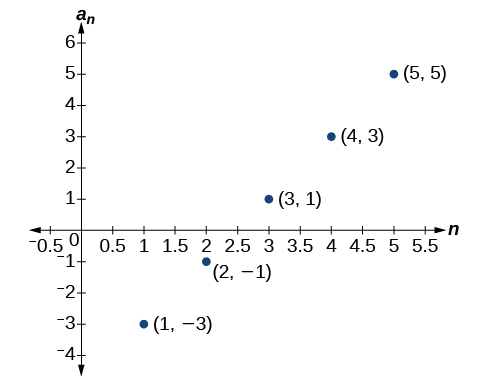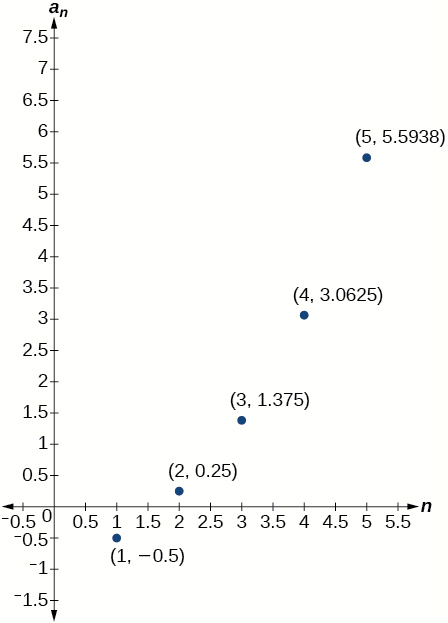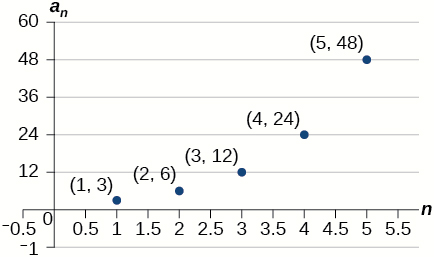# 13.3 Geometric sequences  (Page 4/6)

 Page 4 / 6

## Verbal

What is a geometric sequence?

A sequence in which the ratio between any two consecutive terms is constant.

How is the common ratio of a geometric sequence found?

What is the procedure for determining whether a sequence is geometric?

Divide each term in a sequence by the preceding term. If the resulting quotients are equal, then the sequence is geometric.

What is the difference between an arithmetic sequence and a geometric sequence?

Describe how exponential functions and geometric sequences are similar. How are they different?

Both geometric sequences and exponential functions have a constant ratio. However, their domains are not the same. Exponential functions are defined for all real numbers, and geometric sequences are defined only for positive integers. Another difference is that the base of a geometric sequence (the common ratio) can be negative, but the base of an exponential function must be positive.

## Algebraic

For the following exercises, find the common ratio for the geometric sequence.

$1,3,9,27,81,...$

$-0.125,0.25,-0.5,1,-2,...$

The common ratio is $-2$

$-2,-\frac{1}{2},-\frac{1}{8},-\frac{1}{32},-\frac{1}{128},...$

For the following exercises, determine whether the sequence is geometric. If so, find the common ratio.

$-6,-12,-24,-48,-96,...$

The sequence is geometric. The common ratio is 2.

$5,5.2,5.4,5.6,5.8,...$

$-1,\frac{1}{2},-\frac{1}{4},\frac{1}{8},-\frac{1}{16},...$

The sequence is geometric. The common ratio is $-\frac{1}{2}.$

$6,8,11,15,20,...$

$0.8,4,20,100,500,...$

The sequence is geometric. The common ratio is $5.$

For the following exercises, write the first five terms of the geometric sequence, given the first term and common ratio.

$\begin{array}{cc}{a}_{1}=8,& r=0.3\end{array}$

$\begin{array}{cc}{a}_{1}=5,& r=\frac{1}{5}\end{array}$

$5,1,\frac{1}{5},\frac{1}{25},\frac{1}{125}$

For the following exercises, write the first five terms of the geometric sequence, given any two terms.

$\begin{array}{cc}{a}_{7}=64,& {a}_{10}\end{array}=512$

$\begin{array}{cc}{a}_{6}=25,& {a}_{8}\end{array}=6.25$

$800,400,200,100,50$

For the following exercises, find the specified term for the geometric sequence, given the first term and common ratio.

The first term is $2,$ and the common ratio is $3.$ Find the 5 th term.

The first term is 16 and the common ratio is $-\frac{1}{3}.$ Find the 4 th term.

${a}_{4}=-\frac{16}{27}$

For the following exercises, find the specified term for the geometric sequence, given the first four terms.

${a}_{n}=\left\{-1,2,-4,8,...\right\}.$ Find ${a}_{12}.$

${a}_{n}=\left\{-2,\frac{2}{3},-\frac{2}{9},\frac{2}{27},...\right\}.$ Find ${a}_{7}.$

${a}_{7}=-\frac{2}{729}$

For the following exercises, write the first five terms of the geometric sequence.

$\begin{array}{cc}{a}_{1}=-486,& {a}_{n}=-\frac{1}{3}\end{array}{a}_{n-1}$

$\begin{array}{cc}{a}_{1}=7,& {a}_{n}=0.2{a}_{n-1}\end{array}$

$7,1.4,0.28,0.056,0.0112$

For the following exercises, write a recursive formula for each geometric sequence.

${a}_{n}=\left\{-1,5,-25,125,...\right\}$

${a}_{n}=\left\{-32,-16,-8,-4,...\right\}$

$\begin{array}{cc}a{}_{1}=-32,& {a}_{n}=\frac{1}{2}{a}_{n-1}\end{array}$

${a}_{n}=\left\{14,56,224,896,...\right\}$

${a}_{n}=\left\{10,-3,0.9,-0.27,...\right\}$

$\begin{array}{cc}{a}_{1}=10,& {a}_{n}=-0.3{a}_{n-1}\end{array}$

${a}_{n}=\left\{0.61,1.83,5.49,16.47,...\right\}$

${a}_{n}=\left\{\frac{3}{5},\frac{1}{10},\frac{1}{60},\frac{1}{360},...\right\}$

$\begin{array}{cc}{a}_{1}=\frac{3}{5},& {a}_{n}=\frac{1}{6}{a}_{n-1}\end{array}$

${a}_{n}=\left\{-2,\frac{4}{3},-\frac{8}{9},\frac{16}{27},...\right\}$

${a}_{n}=\left\{\frac{1}{512},-\frac{1}{128},\frac{1}{32},-\frac{1}{8},...\right\}$

${a}_{1}=\frac{1}{512},{a}_{n}=-4{a}_{n-1}$

For the following exercises, write the first five terms of the geometric sequence.

${a}_{n}=-4\cdot {5}^{n-1}$

${a}_{n}=12\cdot {\left(-\frac{1}{2}\right)}^{n-1}$

$12,-6,3,-\frac{3}{2},\frac{3}{4}$

For the following exercises, write an explicit formula for each geometric sequence.

${a}_{n}=\left\{-2,-4,-8,-16,...\right\}$

${a}_{n}=\left\{1,3,9,27,...\right\}$

${a}_{n}={3}^{n-1}$

${a}_{n}=\left\{-4,-12,-36,-108,...\right\}$

${a}_{n}=\left\{0.8,-4,20,-100,...\right\}$

${a}_{n}=0.8\cdot {\left(-5\right)}^{n-1}$

${a}_{n}=\left\{-1.25,-5,-20,-80,...\right\}$

${a}_{n}=\left\{-1,-\frac{4}{5},-\frac{16}{25},-\frac{64}{125},...\right\}$

${a}_{n}=-{\left(\frac{4}{5}\right)}^{n-1}$

${a}_{n}=\left\{2,\frac{1}{3},\frac{1}{18},\frac{1}{108},...\right\}$

${a}_{n}=\left\{3,-1,\frac{1}{3},-\frac{1}{9},...\right\}$

${a}_{n}=3\cdot {\left(-\frac{1}{3}\right)}^{n-1}$

For the following exercises, find the specified term for the geometric sequence given.

Let ${a}_{1}=4,$ ${a}_{n}=-3{a}_{n-1}.$ Find ${a}_{8}.$

Let ${a}_{n}=-{\left(-\frac{1}{3}\right)}^{n-1}.$ Find ${a}_{12}.$

${a}_{12}=\frac{1}{177,147}$

For the following exercises, find the number of terms in the given finite geometric sequence.

${a}_{n}=\left\{-1,3,-9,...,2187\right\}$

${a}_{n}=\left\{2,1,\frac{1}{2},...,\frac{1}{1024}\right\}$

There are $12$ terms in the sequence.

## Graphical

For the following exercises, determine whether the graph shown represents a geometric sequence.The graph does not represent a geometric sequence.

For the following exercises, use the information provided to graph the first five terms of the geometric sequence.

$\begin{array}{cc}{a}_{1}=1,& r=\frac{1}{2}\end{array}$

$\begin{array}{cc}{a}_{1}=3,& {a}_{n}=2{a}_{n-1}\end{array}$${a}_{n}=27\cdot {0.3}^{n-1}$

## Extensions

Use recursive formulas to give two examples of geometric sequences whose 3 rd terms are $\text{\hspace{0.17em}}200.$

Answers will vary. Examples: ${\begin{array}{cc}{a}_{1}=800,& {a}_{n}=0.5a\end{array}}_{n-1}$ and ${\begin{array}{cc}{a}_{1}=12.5,& {a}_{n}=4a\end{array}}_{n-1}$

Use explicit formulas to give two examples of geometric sequences whose 7 th terms are $1024.$

Find the 5 th term of the geometric sequence $\left\{b,4b,16b,...\right\}.$

${a}_{5}=256b$

Find the 7 th term of the geometric sequence $\left\{64a\left(-b\right),32a\left(-3b\right),16a\left(-9b\right),...\right\}.$

At which term does the sequence exceed $100?$

The sequence exceeds $100$ at the 14 th term, ${a}_{14}\approx 107.$

At which term does the sequence begin to have integer values?

For which term does the geometric sequence ${a}_{{}_{n}}=-36{\left(\frac{2}{3}\right)}^{n-1}$ first have a non-integer value?

${a}_{4}=-\frac{32}{3}\text{\hspace{0.17em}}$ is the first non-integer value

Use the recursive formula to write a geometric sequence whose common ratio is an integer. Show the first four terms, and then find the 10 th term.

Use the explicit formula to write a geometric sequence whose common ratio is a decimal number between 0 and 1. Show the first 4 terms, and then find the 8 th term.

Answers will vary. Example: Explicit formula with a decimal common ratio: ${a}_{n}=400\cdot {0.5}^{n-1};$ First 4 terms: $\begin{array}{cc}400,200,100,50;& {a}_{8}=3.125\end{array}$

Is it possible for a sequence to be both arithmetic and geometric? If so, give an example.

Find the possible value of 8.5 using moivre's theorem
which of these functions is not uniformly cintinuous on (0, 1)? sinx
which of these functions is not uniformly continuous on 0,1
solve this equation by completing the square 3x-4x-7=0
X=7
Muustapha
=7
mantu
x=7
mantu
3x-4x-7=0 -x=7 x=-7
Kr
x=-7
mantu
9x-16x-49=0 -7x=49 -x=7 x=7
mantu
what's the formula
Modress
-x=7
Modress
new member
siame
what is trigonometry
deals with circles, angles, and triangles. Usually in the form of Soh cah toa or sine, cosine, and tangent
Thomas
solve for me this equational y=2-x
what are you solving for
Alex
solve x
Rubben
you would move everything to the other side leaving x by itself. subtract 2 and divide -1.
Nikki
then I got x=-2
Rubben
it will b -y+2=x
Alex
goodness. I'm sorry. I will let Alex take the wheel.
Nikki
ouky thanks braa
Rubben
I think he drive me safe
Rubben
how to get 8 trigonometric function of tanA=0.5, given SinA=5/13? Can you help me?m
More example of algebra and trigo
What is Indices
If one side only of a triangle is given is it possible to solve for the unkown two sides?
cool
Rubben
kya
Khushnama
please I need help in maths
Okey tell me, what's your problem is?
Navin
the least possible degree ?
(1+cosA)(1-cosA)=sin^2A
good
Neha
why I'm sending you solved question
Mirza
Teach me abt the echelon method
Khamis
exact value of cos(π/3-π/4)
What is differentiation?ByByBy Subramanian DivyaBy Rhodes